Custom SearchPOWERS OF FUNCTIONS Theorem 6. The derivative of any differentiable function of x raised to the power n, where n is any real number, is equal to n times the polynomial function of x to the (n - 1) power times the derivative of the polynomial itself. Ifwhere u is any differentiable function of x, then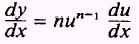EXAMPLE. Find the derivative of the functionSOLUTION.- Apply Theorem 6 and find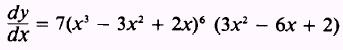EXAMPLE. Find the derivative of the function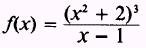SOL UTION.- This problem involves Theorem 5 and Theorem 6. Theorem 6 is used to find the derivative of the numerator; then Theorem 5 is used to find the derivative of the resulting quotient. The derivative of the numerator is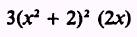and the derivative of the denominator is 1. Then, by Theorem 5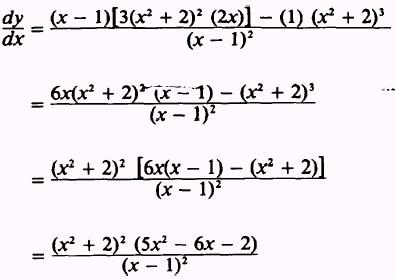PRACTICE PROBLEMS: Find the derivatives of the following:ANSWERS: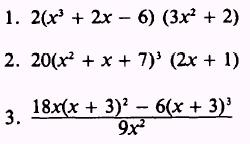Integrated Publishing, Inc. - A (SDVOSB) Service Disabled Veteran Owned Small Business